# Python数据分析教程（一）：Numpy

## 数据的纬度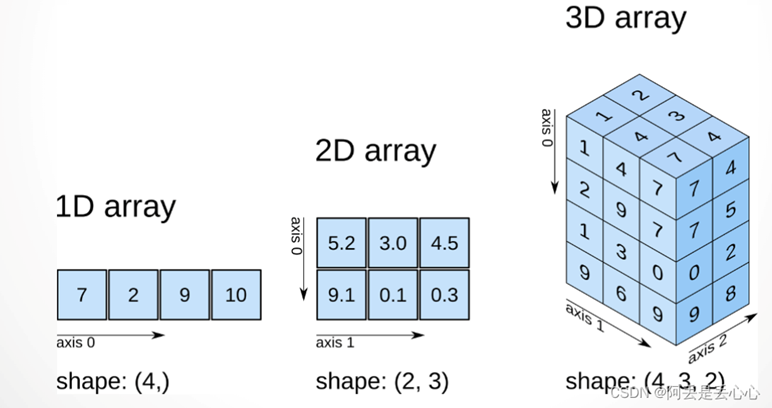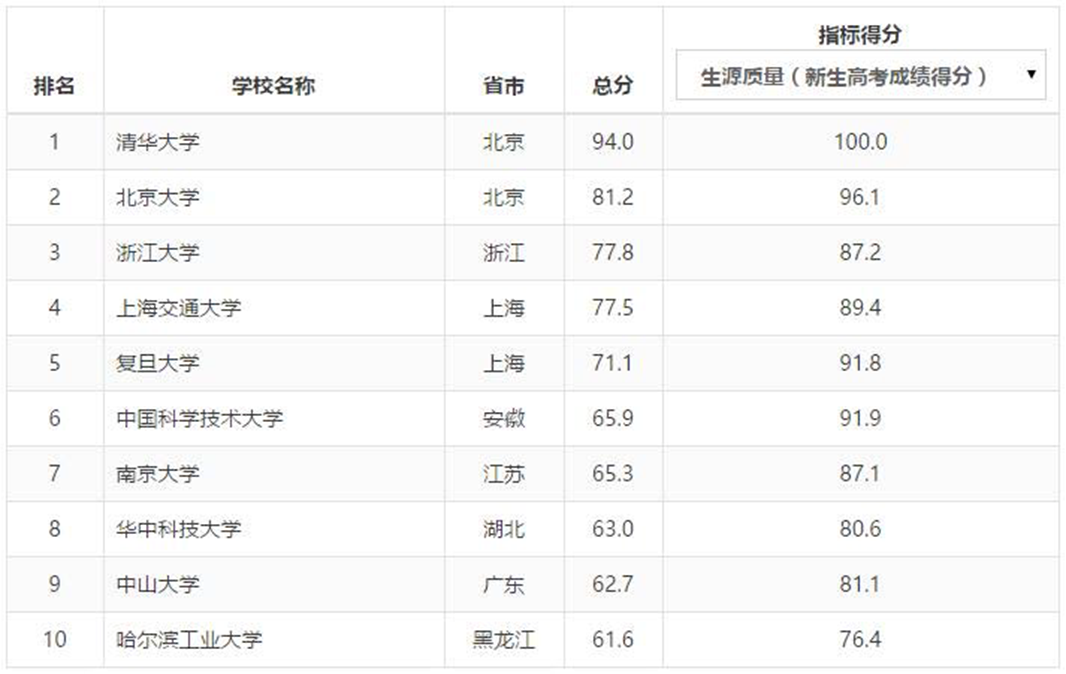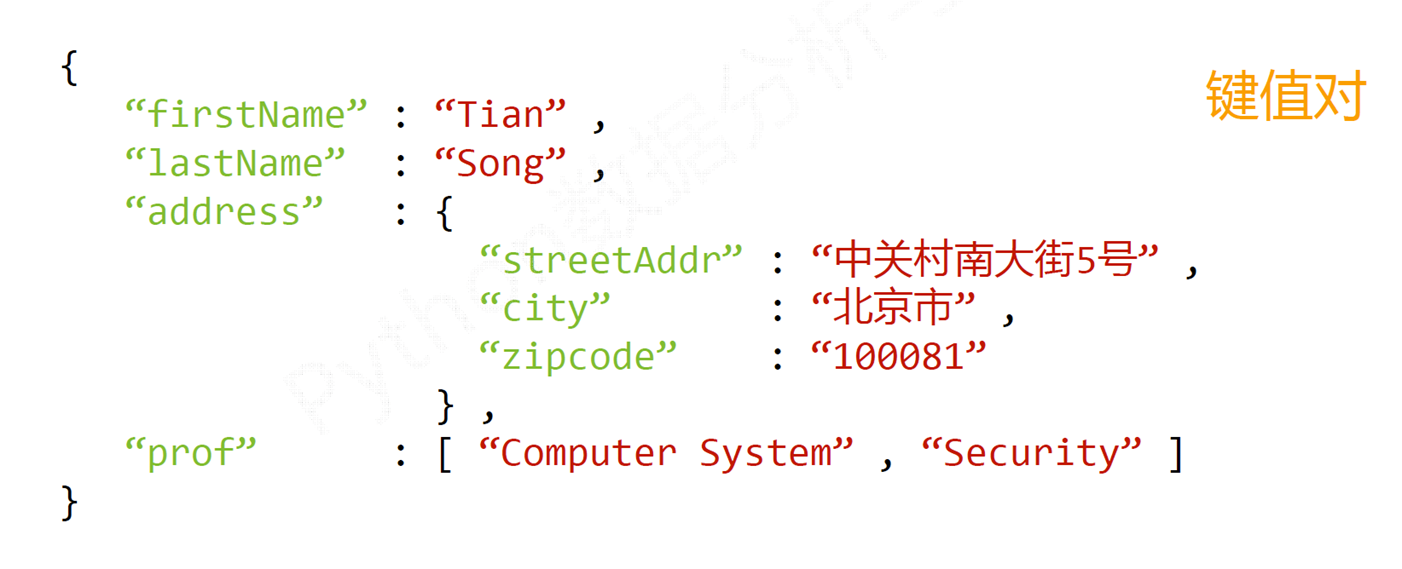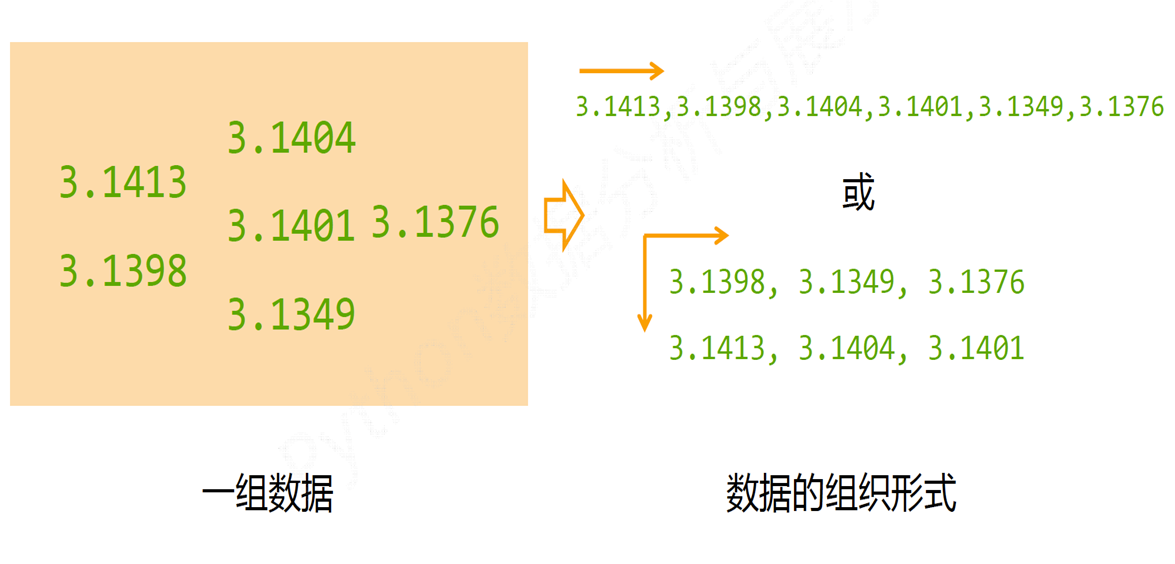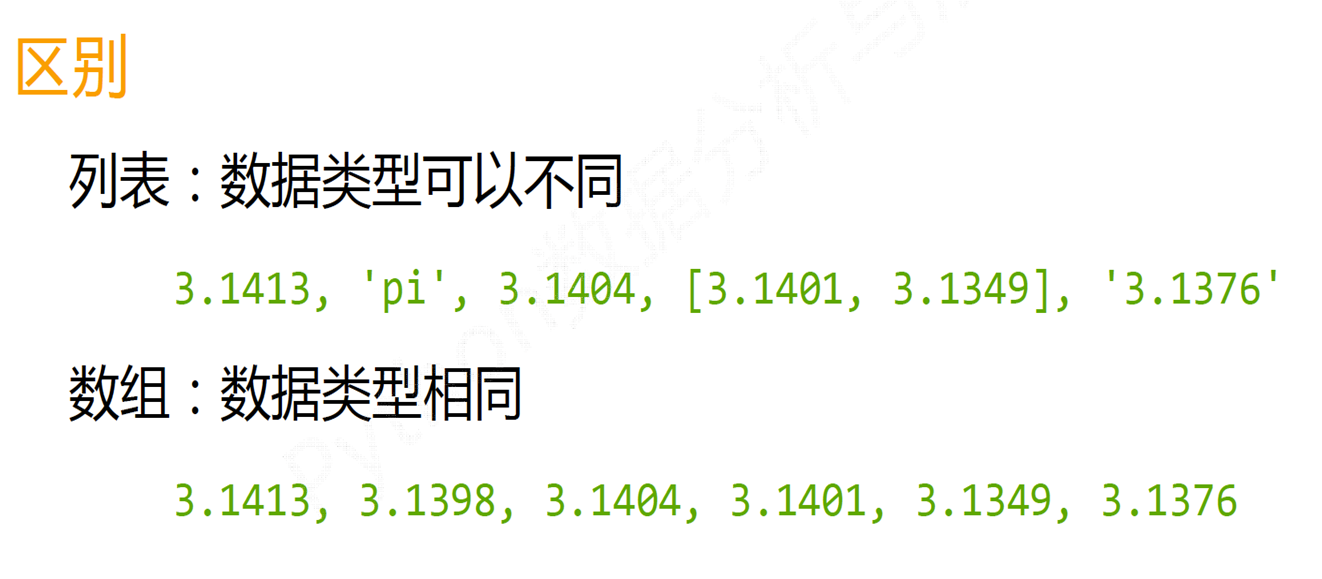## Numpy

### Numpy介绍

• NumPy是一个开源的Python科学计算基础库，包含：

• 一个强大的N维数组对象ndarray

• 广播功能函数

• 整合C/C++/Fortran代码的工具

• 线性代数、傅里叶变换、随机数生成等功能

• NumPy是SciPy、Pandas等数据处理或科学计算库的基础

``import numpy as np``

### N维数组对象：ndarray

• 数组对象可以去掉元素间运算所需的循环，使一维向量更像单个数据

• 设置专门的数组对象，经过优化，可以提升这类应用的运算速度————科学计算中，一个维度所有数据的类型往往相同

• 数组对象采用相同的数据类型，有助于节省运算和存储空间### ndarray实例

• ndarray是一个多维数组对象，由两部分构成：
• 实际的数据
• 描述这些数据的元数据（数据维度、数据类型等）
• ndarray数组一般要求所有元素类型相同（同质），数组下标从0开始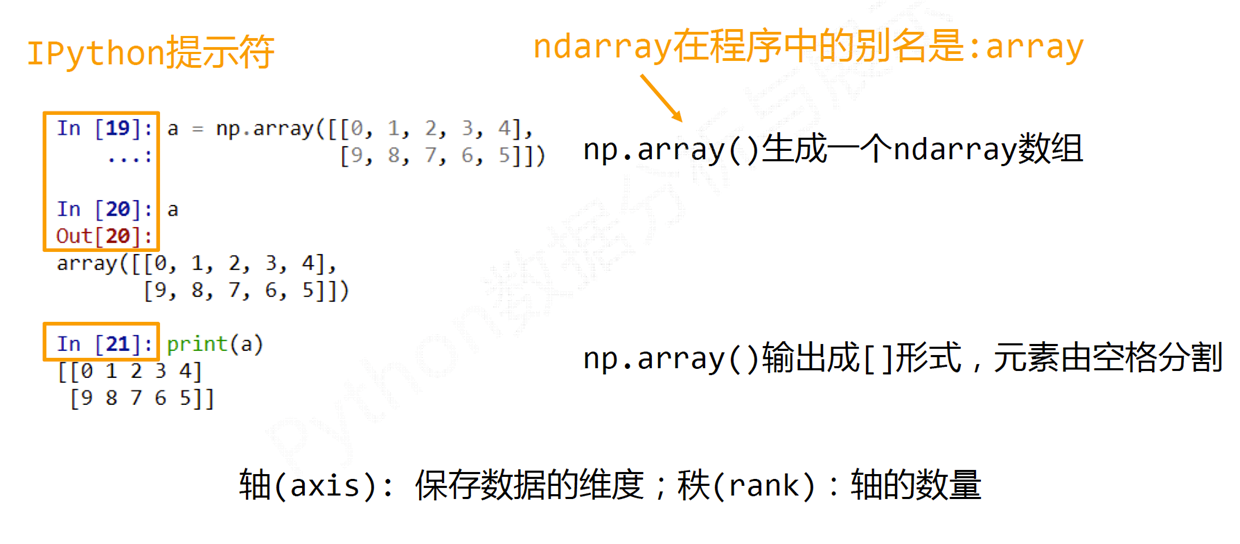### ndarray对象的属性

``````#属性&说明
.ndim #秩，即轴的数量或维度的数量
.shape #ndarray对象的尺度，对于矩阵，n行m列
.size #ndarray对象元素的个数，相当于.shape中n*m的值
.dtype #ndarray对象的元素类型
.itemsize #ndarray对象中每个元素的大小，以字节为单位``````#### ndarray的元素类型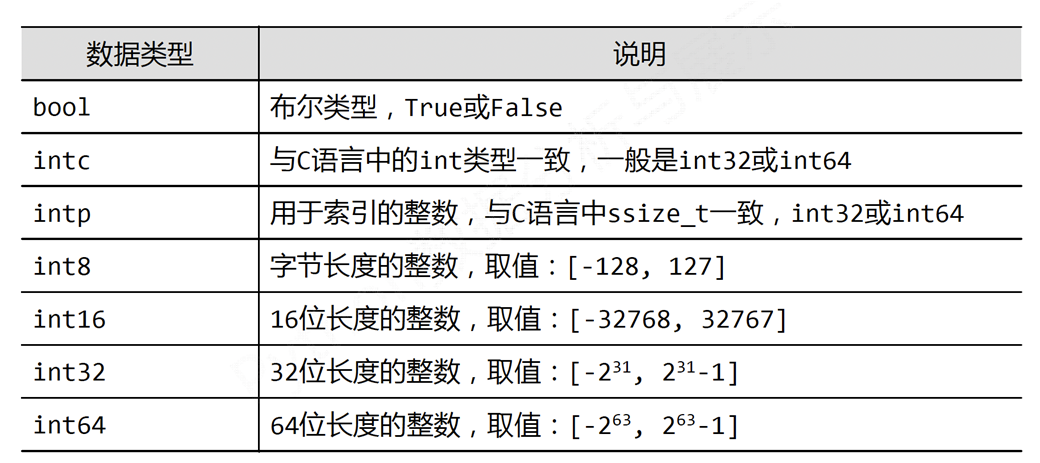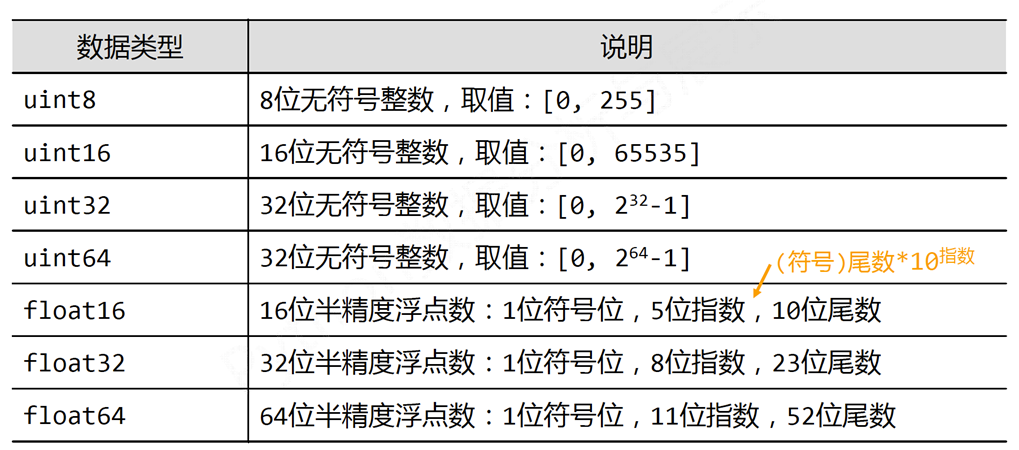### ndarray数组的创建方法

• 从Python中的列表、元组等类型创建ndarray数组
• 使用NumPy中函数创建ndarray数组，如：arange, ones, zeros等
• 从字节流（raw bytes）中创建ndarray数组
• 从文件中读取特定格式，创建ndarray数组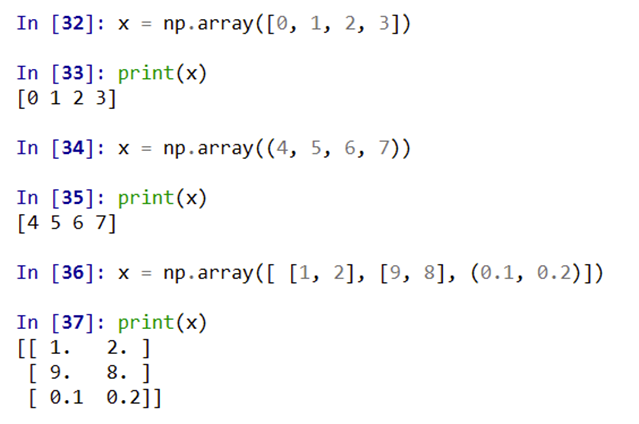``````#函数&说明
np.arange(n) #类似range()函数，返回ndarray类型，元素从0到n-1
np.ones(shape) #根据shapes生成一个全l数组，shape是元组类型
np.zeros(shape) #根据shape生成一个全数组，shape是元组类型
np.full(shape,val) #根据shape:生成一个数组，每个元素值都是val
np.eye(n) #创建一个正方的n*n单位矩阵，对角线为1，其余为0``````

ndarray数组的变换:

``````#方法&说明
.reshape(shape) #不改变数组元素，返回一个shape形状的数组，原数组不变
.resize(shape) #与.reshape()功能一致，但修改原数组
.swapaxes(ax1,ax2) #将数组n个维度中两个维度进行调换
.flatten() #对数组进行降维，返回折叠后的一维数组，原数组不变``````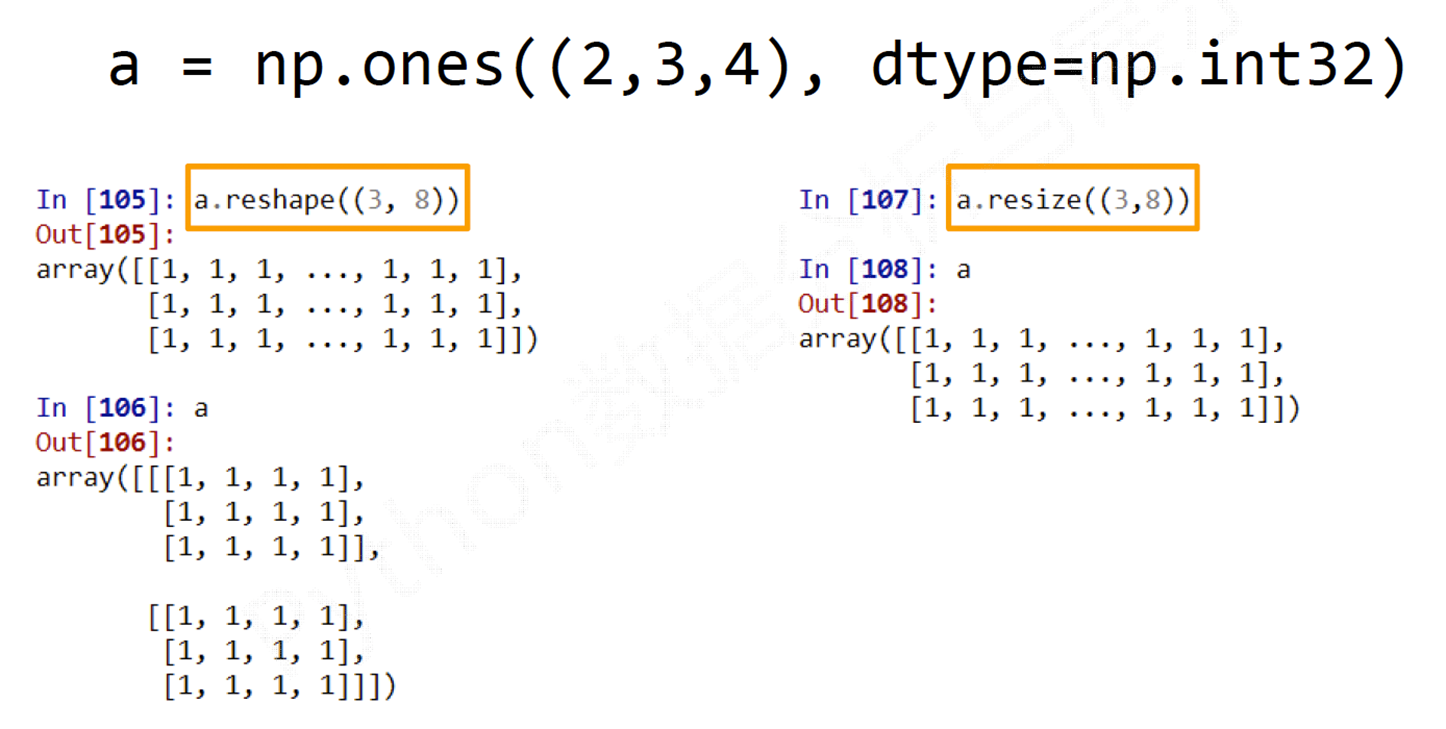• 索引：获取数组中特定位置元素的过程
• 切片：获取数组元素子集的过程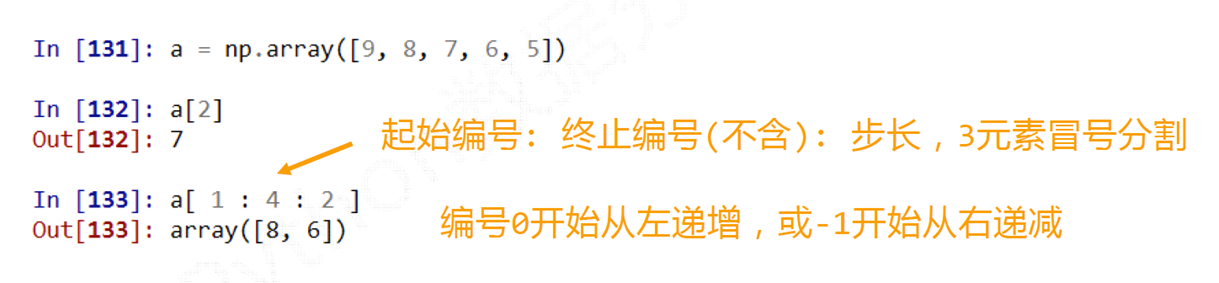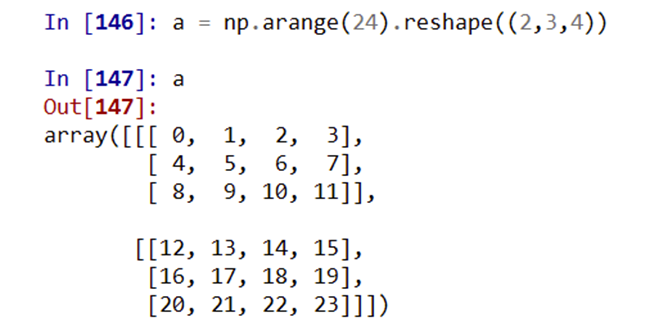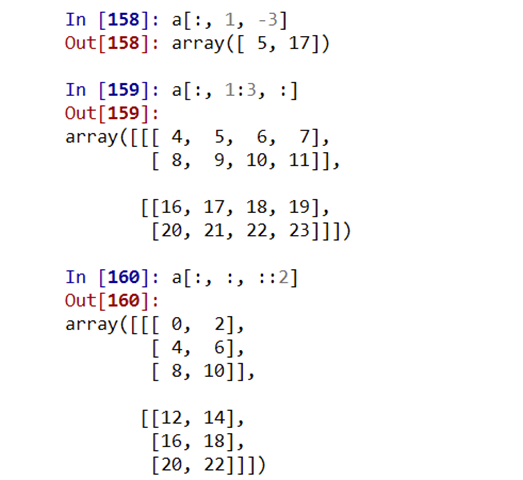### ndarray数组的运算

NumPy一元函数:

``````#函数&说明
np.abs(x)
np.fabs(x)#计算数组各元素的绝对值
np.sqrt(x)#计算数组各元素的平方根
np.square(x)#计算数组各元素的平方
np.log(x)
np.1og10(x)
np.1og2(x)#计算数组各元素的自然对数、10底对数和2底对数
np.ceil(x)
np.floor(x)#计算数组各元素的ceiling值或f1oor值
np.rint(x)#计算数组各元素的四舍五入值
np.modf(x)#将数组各元素的小数和整数部分以两个独立数组形式返回
np.cos(x)np.cosh(x)
np.sin(x)np.sinh(x)#计算数组各元素的普通型和双曲型三角函数
np.tan(x)np.tanh(x)
np.exp(x)#计算数组各元素的指数值
np.sign(x)#计算数组各元素的符号值，1(+)，0，-1(-)``````

NumPy二元函数:

``````#函数&说明
+ - * / ** #两个数组各元素进行对应运算
np.maximum(x,y)
np.fmax()
np.minimum(x,y)
np.fmin() #元素级的最大值/最小值计算
np.mod(x,y) #元素级的模运算
np.copysign(x,y) #将数组y中各元素值的符号赋值给数组x对应元素
>< >= <= == != #算术比较，产生布尔型数组``````

Numpy数据存取:

csv格式:``np.savetxt(frame,array,fmt='%.18e',delimiter=None)``
• frame：文件、字符串或产生器，可以是.gz或.bz2的压缩文件
• array:存入文件的数组
• fmt:写入文件的格式，例如：%d%.2f%.18e
• delimiter:分割字符串，默认是任何空格

``````np.loadtxt(frame,dtype=np.float,delimiter=None,
unpack=False)``````
• frame:文件、字符串或产生器，可以是.gz或.bz2的压缩文件
• dtype:数据类型，可选
• delimiter:分割字符串，默认是任何空格
• unpack:如果True,读入属性将分别写入不同变量

``````a.tofile(frame, sep='', format='%s')
np.fromfile(frame, dtype=float, count=‐1, sep='')``````

numpy随机数函数子库:

``np.random.*``
``````#函数&说明
rand(d0,d1,..,dn) #根据d0-dn创建随机数数组，浮点数，[0,1)，均匀分布
randn(d0,d1,..,dn) #根据d0-dn创建随机数数组，标准正态分布
randint(low[,high,shape]) #根据shapet创建随机整数或整数数组，范围是[low,high)
seed(s) #随机数种子，s是给定的种子值
shuffle(a) #根据数组a的第1轴进行随排列，改变数组×
permutation(a) #根据数组a的第1轴产生一个新的乱序数组，不改变数组x
choice(a[,size,replace,p]) #从一维数组a中以概率p抽取元素，形成size形状新数组 replace表示是否可以重用元素，默认为False
uniform(low,high,size) #产生具有均匀分布的数组，low起始值，high结束值，size形状
normal(loc,scale,size) #产生具有正态分布的数组，loc均值，scale标准差，size形状
poisson(lam,size) #产生具有泊松分布的数组，lam随机事件发生率，size形状``````

numpy统计函数:

``np.*``
``````#函数&说明
sum(a,axis=None) #根据给定轴axis计算数组a相关元素之和，axis整数或元组
mean(a,axis=None) #根据给定轴axis计算数组a相关元素的期望，axis整数或元组
average(a,axis=None,weights=None) #根据给定轴axis计算数组a相关元素的加权平均值
std(a,axis=None) #根据给定轴axis计算数组a相关元素的标准差
var(a,axis=None) #根据给定轴axis计算数组a相关元素的方差
min(a)
max(a) #计算数组a中元素的最小值、最大值
argmin(a)
argmax(a) #计算数组a中元素最小值、最大值的降一维后下标
unravel_index(index,shape) #根据shape?将一维下标index转换成多维下标
ptp(a) #计算数组a中元素最大值与最小值的差
median(a) #计算数组a中元素的中位数（中值）``````

numpy替换函数:

• np.where(condition, x, y) ——满足条件(condition)，输出x，不满足输出y。
• 只有条件 (condition)，没有x和y，则输出满足条件 (即非0) 元素的坐标 (等价于numpy.nonzero)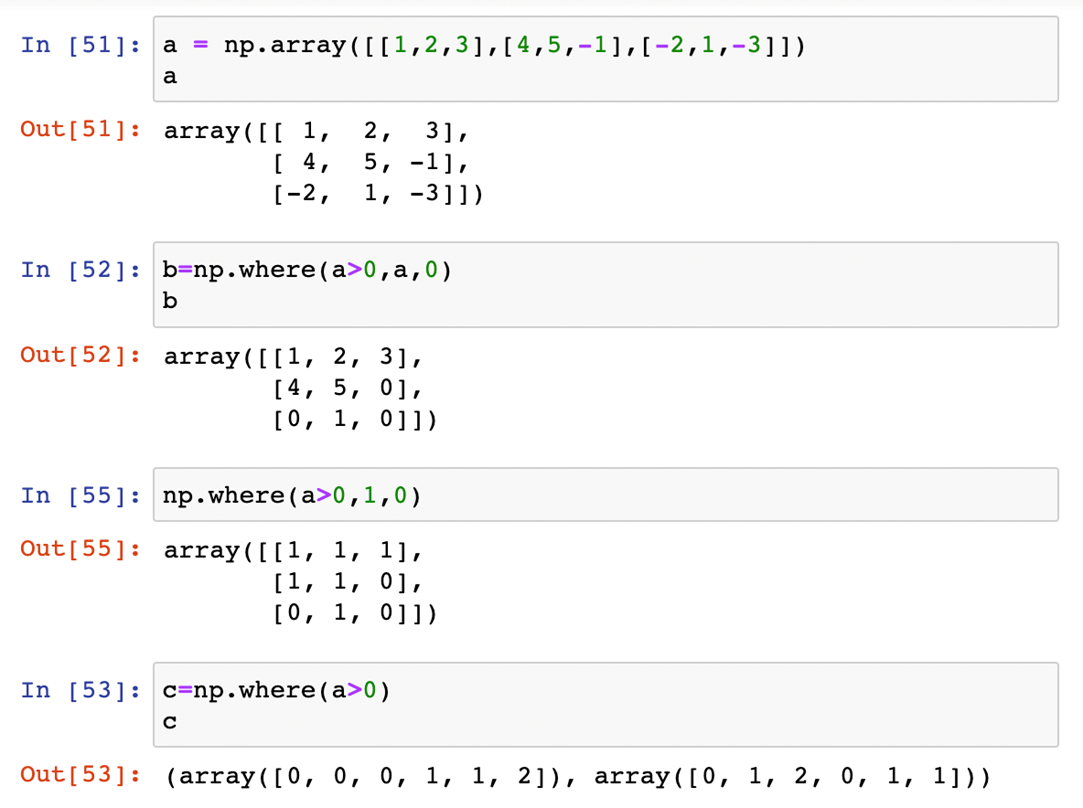### numpy数据存取与函数**

CSV文件

``````np.loadtxt()
np.savetxt()``````

``````a.tofile()
np.fromfile()
np.save()
np.savez()

``````np.random.rand()
np.random.randn()
np.random.randint()
np.random.seed()
np.random.shuffle()
np.random.permutation()
np.random.choice()``````

目录